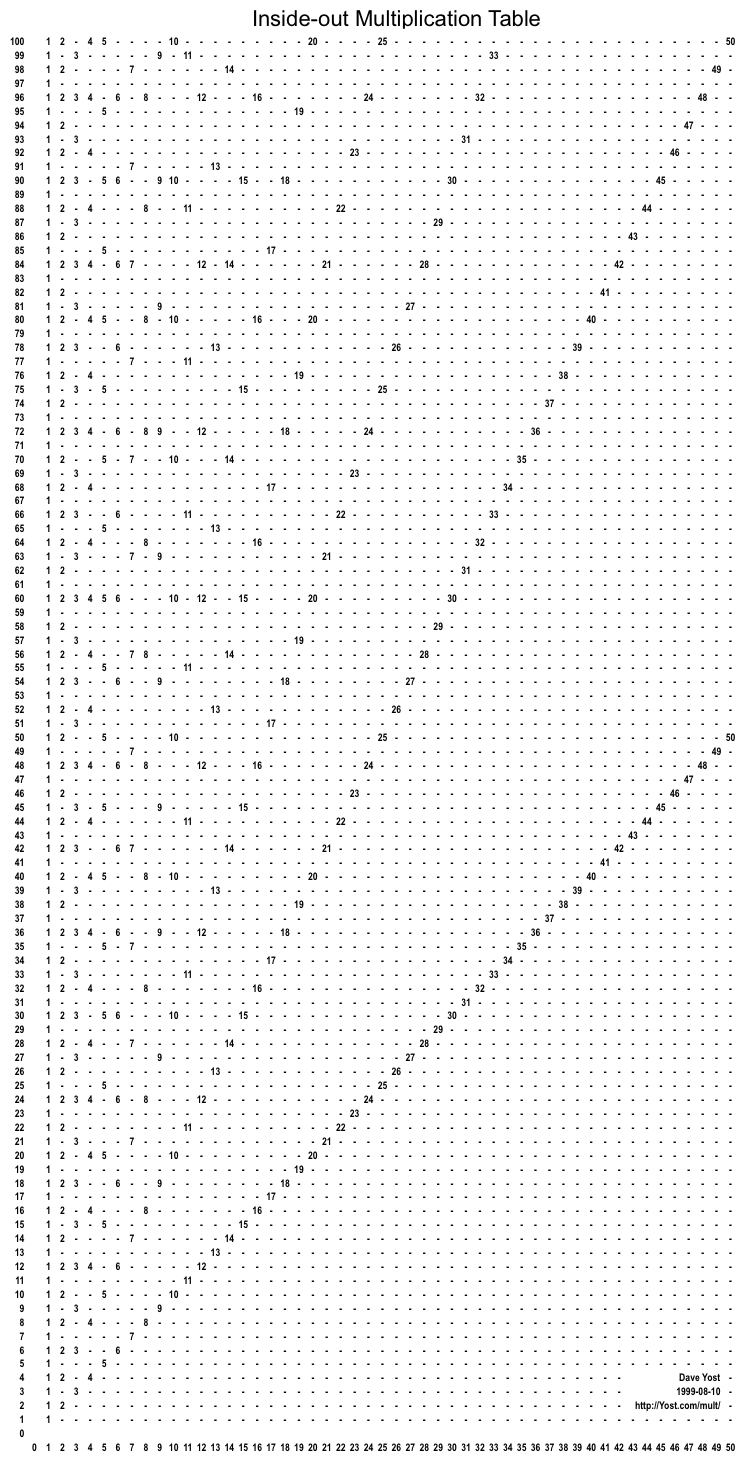## Inside-out Multiplication Table

### Here's a new(?) way to look at the multiplication table.

The table is a grid of cells, each containing either a number or a "-" for a place-holder.

Each cell in the grid is in a vertical column and a horizontal row. The column numbers are across the bottom, and the row numbers are on the left side. The cell in column 3, row 9 is called {3,9}.

If the number of a row is divisible by 2, then the cell in column 2 of that row has a "2" in it, otherwise just "-". The same for 3, 4, 5, etc. So, the numbers in a row are the row number's factors. A cell with a number in it is called a "numbered cell".The image on this page won't print well. Use one of the links below for printing.

(To really get it, you should download a file below and print out a few copies to mark up with a pencil on paper.)

You can see the numbered cells forming lines radiating diagonally up from the lower-left. What can you notice about those lines? Draw in the lines by connecting the numbers.

In a regular muliplication table, you locate the row and column numbers of the two numbers you want to multiply together, and the answer is in the cell that belongs to them. In this table the row numbers are the answers(!), and one of the numbers to be multiplied is the column number. How do you locate the other number to be multiplied? Can you see why I call it the Inside-out Multiplication Table? Other names could be Convoluted Multiplicaton Table or Factor Table.

Look at row 60. See all the factors it has? Nice number to use for something you'll divide up a lot, like the number of minutes in an hour.

It's easy to find prime (row) numbers. They're the ones with only themselves and 1 for factors.

You can connect groups of numbered cells together into some curves. Do you see any? Each curve passes through numbers in the sequence 0, 1, 2, 3, 4, 5, ... Draw at least some of the curve that has {10,100} (the 10 on the top line) as the peak of the curve and that passes through the nearby 8, 9, 11, and 12. Draw another curve that starts at {0,0}, then goes through {1,1}, {2,4}, {3,9}, {4,16}, etc. This curve passes through all of the squares. It's especially interesting because all the cells to its left are less than the square root of the row, and all the cells to its right are greater than the square root of the row.

Each of the two curves you just drew belongs to one of two families of curves. Each numbered cell is on one curve in each family, except for {0,0}, which is on every curve in both families. These two families of curves make a really warped grid. See if you can connect the numbers to draw in some of the curves.